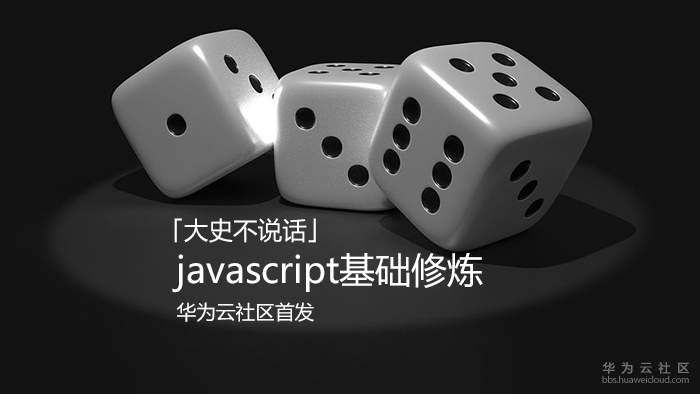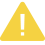# javascript基础修炼（8）——指向FP世界的箭头函数

【摘要】 一. 箭头函数箭头函数是ES6语法中加入的新特性，而它也是许多开发者对ES6仅有的了解，每当面试里被问到关于“ES6里添加了哪些新特性？”这种问题的时候，几乎总是会拿箭头函数来应付。箭头函数，=&gt;，没有自己的this , arguments , super , new.target ，“书写简便，没有this”在很长一段时间内涵盖了大多数开发者对于箭头函数的全部认知（当然也包括我自己）...## 二. 更贴近本能的思维方式`let resolveYX = (x) => 3*x*x + 2*x + 1;let resolveZY = (y) => 4*y*y*y + 5*y*y + 6;let resolveRZ = (z) => (2*z*z - 4)/3;let y = resolveYX（2）；let z = resolveZY(y);let result = resolveRZ(z);`## 三. 函数式编程

### 3.1 传统代码的实现

```function Task(value){    this.value = value;
}

}

### 3.2 函数式代码推演

【函数式编程】，我们不再采用程序语言按照步骤来复现一个业务逻辑，而是换一个更为抽象的角度，用数学的眼光看待所发生的事情。那么上面的代码实际上所做的事情就是：

\$\$
y=f(x)
\$\$

```function prepare(){    return function (x){        return (new Task(x)).step(fn1).step(fn2).step(fn3);
}
}let f = prepare();let y = f(x);```

`//fn2Result是XX.step(fn2)执行完后返回的结果(值和方法都包含在Task容器中)fn2Result.step(fn3);`

```//先生成一个用于生成新函数的高阶函数，来实现局部调用let goStep = function(fn){    return function(params){        let value = fn(params.value);        return new Task(value);
}
}//fn2Result.step(fn3)这一句将被转换为如下形式let requireFn2Result = goStep(fn3);```

```function prepare(){    return function (x){        let fn2Result = (new Task(x)).step(fn1).step(fn2);
return requireFn2Result(fn2Result);
}
}```

```//暂时先忽略函数声明的位置let requireFn2Result = goStep(fn3);let requireFn1Result = goStep(fn2);let requireInitResult = goStep(fn1);function prepare(){    return function (x){        let InitResult = new Task(x);        return requireFn2Result(requireFn1Result(requireInitResult(InitResult)));
}
}```

```let createTask = function(x){    return new Task(x);
};```

### 3.3 函数化的代码

```function prepare(){    return function (x){        return requireFn2Result(requireFn1Result(requireInitResult(createTask(x))));
}
}let f = prepare();let y = f(x);```

### 3.5 进一步抽象

3.3节中函数化的代码中，存在一个很长的嵌套调用，如果业务逻辑步骤过多，那么这行代码会变得很长，同时也很难阅读，我们需要通过一些手段将这些中间环节的函数展开为一种扁平化的写法。

```/**
*定义一个工具函数compose,接受两个函数作为参数，返回一个新函数
*新函数接受一个x作为入参，然后实现函数的迭代调用。
*/var compose = function (f, g) {    return function (x) {        return f(g(x));
}
};/**
*升级版本的compose函数，接受一组函数，实现左侧函数包裹右侧函数的形态
*/let composeEx = function (...args) {    return (x)=>args.reduceRight((pre,cur)=>cur(pre),x);
}```

```let pipeline = composeEx(requireFn2Result,requireFn1Result,requireInitResult,createTask);function prepare(){    return function (x){        return pipeline(x);
}
}let f = prepare();let y = f(x);```

`//prepare执行后得到的新函数let f = x => pipeline(x);`

`let f = pipeline;`

### 3.6 完整的转换代码

```let composeEx = (...args) => (x) => args.reduceRight((pre,cur) =>cur(pre),x);let getValue = (obj) => obj.value;let createTask = (x) => new Task(x);/*goStep执行后得到的函数也满足前面提到的“let f=(x)=>g(x)”的形式，可以将其pointfree化.
let goStep = (fn)=>(params)=>composeEx(createTask, fn, getValue)(params);
let requireFn2Result = goStep(fn3);

## 四. 两个主角

`程序设计 = 数据结构 + 算法   Vs   函数式编程 = 数据 + 函数`

### 函数Vs纯函数

• 只依赖自己的参数

• 执行过程没有副作用

```var a = 1;function inc(x){    return a + x;
}function pureInc(x){    let a = 1;    return x + a;
}```

### 数据Vs不可变数据

`javascript`中的对象很强大也很灵活，可并不是所有的场景中我们都需要这种灵活性。来看这样一个例子：

```let a = {    name:'tony'}let b = a;
modify(b);console.log(a.name);```

【深拷贝】是一种典型的防御性编程，因为在浅拷贝的机制下，修改对象属性的时候会影响到所有指向它的标识符，从而造成不可预测的结果。

`javascript`中，常见的深拷贝都是通过递归来实现的，然后利用语言特性做出一些代码层面的优化，例如各个第三方库中的`extend( )`方法或者`deepClone( )`。可是当一个结构很深或者复杂度很高时，深拷贝的耗时就会大幅增加，有的时候我们关注的可能只是数据结构中的一部分，也就是说新老对象中很大一部分数据是一致的，可以共享的，但深拷贝过程中忽视了这种情况而简单粗暴地对整个对象进行递归遍历和克隆。

```//Immutable.js官网示例
var map1 = Immutable.Map({ a: 1, b: 2, c: 3 }); var map2 = map1.set('b', 50);
map1.get('b'); // 2
map2.get('b'); // 50```

## 四. 前端的学习路线

• javascript基础

如果你能够很清楚`高阶函数``柯里化``反柯里化`这些关键词的含义和一般用途，并且至少了解`Array``map``reduce`方法做了什么事情，那么就可以进行下一步。否则就需要好好复习一下`javascript`的基础知识。在`javascript`中进行函数式编程会反复涉及到这些基本技术的运用。

• 《javascript函数式编程指南》

这是一本来自于gitbook的翻译版的非常棒的开源电子书，这本书很棒，但是如果将函数式编程的相关知识分为初中高级的话，这本书似乎只涵盖了初级和高级，而省略了中级的部分，当内容涉及到范畴论和代数结构的时候，理解难度会突然一下变得很大。当你读不懂的时候可以先停下来，用下一个资料进行过渡，然后回过头来再继续阅读后续的部分。

同时提一句，翻译者@胡子大哈也是之前提及的那本著名的《React小书》的主要作者。

• Ramda.js官网博文集

`Ramda.js``javascript`提供了一系列函数式编程的工具函数，但官网的《Thinking In Ramda》系列教程，是非常好的中级教程，结合`Ramda`的API进行讲解，让开发者更容易理解函数式编程，它正好弥补了前一个资料中没有中级教程的问题。

• Ramda.js的API

不得不说很多前端开发者都是从API开始学习函数式编程的，但很快就会发现学了和没学差不多，因为没有理论基础，你很难知道该去使用它。就好像给了你最顶尖的工具，你也没法一次性就做出好吃的牛排，因为你不会做。

• Rx.js和Immutable.js

事实上笔者自己也还没有进行到这个阶段的学习，`Rx.js`是隶属于`Angular`全家桶的，`Immutable.js`是隶属于`React`全家桶的，即使在自己目前的工作中没有直接使用到，你也应该了解它们。

• 代数结构的理论基础

当你具备了基本的使用能力，想要更上一层楼的时候，就需要重新整合函数式编程的理论体系。这个项目用于解释函数式编程的理论基础中各类术语及相关用途。

## 五. 小结

【函数式编程】为我们展现了`javascript`语言的另一种灵活性。

【函数式编程】让开发者理解程序设计这件事本质是是一种设计，是一种创造行为，和其他通过组合功能单元而得到更强大的功能单元的行为没有本质区别。

【版权声明】本文为华为云社区用户原创内容，转载时必须标注文章的来源（华为云社区），文章链接，文章作者等基本信息，否则作者和本社区有权追究责任。如果您发现本社区中有涉嫌抄袭的内容，欢迎发送邮件至：huaweicloud.bbs@huawei.com进行举报，并提供相关证据，一经查实，本社区将立刻删除涉嫌侵权内容。

### 评论 (0)

###### 温馨提示